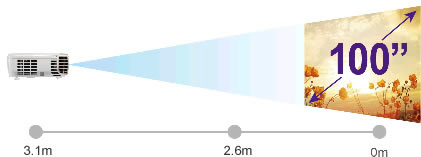# Projector Blog : How to calculate Projector Throw Distance?

##### What is Throw?

Simply put, the ‘Throw’ of a projector is the distance the projector is from the screen. The larger the image is, the further back the projector must be. The exact distance (or Throw) will vary depending on the type of lens used on the projector as well as the amount of zoom included. Projector manufacturers provide a 'Throw Ratio' to allow us to easily calculate the distance the projector must be for a particular image size, we can also work out what image size we would get from a certain distance.

##### How to calculate projector throw distance (or installation point)

Finding the installation point is important to make sure you do not wire up a projector in the wrong position. Every projector comes with a number called the 'throw ratio', which is used to calculate the installation position of the projector. Many projectors will have 2 Throw Ratio's, one for when zoomed in and another for when zoomed out. Using these 2 numbers you can calculate the minimum and maximum distance where you need to place your projector in order to display a certain image size.

The throw ratio will have a number, which represents distance, followed by a colon then a 1, which represents image width. For example, if the throw ratio is 1.15:1, this means if the image width is 1 metre, the distance is 1.15 metres. We can easily use this ratio to calculate the distance based on different image widths. For example, if you have a 2 metre wide image it would mean the installation point is 2.3 metres (2 metre width x 1.15 ratio = 2.3m)

In the event 2 throw ratios are provided (to indicate when zoomed in and zoomed out) you just need to do the calculation twice. If the advertised throw ratio is "1.15 - 1.5:1", and you wish to display a 100" 16:9 image (which is 2.13m wide), then the calculations would look like this:

1.15 x 2.13m = 2.45 metres - Closest installation point

1.50 x 2.13m = 3.20 metres - Furthest installation point

If we install this projector between 2.45 and 3.20m back we'll be able to zoom the picture to fit the required 100" screen. If we place the projector close than 2.45m the image would be smaller, even if we fully zoom the image out.